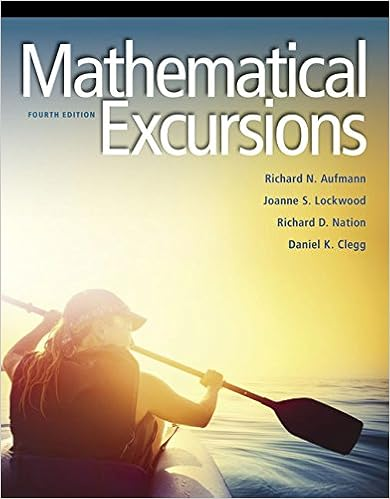# geometry-review-triangle-test.pdf - Unit 4 Test Review...

• Notes
• 6

This preview shows page 1 - 3 out of 6 pages.

##### We have textbook solutions for you!
The document you are viewing contains questions related to this textbook.The document you are viewing contains questions related to this textbook.
Chapter 7 / Exercise 29
Mathematical Excursions
AufmannExpert Verified
Unit 4 Test Review N_________________ Geometry D___________B_____ 4.1Classifying Triangles Name the 6 ways we classify triangles. Give at least 3 examples to show the different classifications. Exterior Angle Theorem: solve for x using exterior angle theorem: 5x + 12 4.2Applying Congruence 1.Two figures are congruent if they have the same ___________________ and _______________________. 2.What is a congruence statement? What can it help us do? 3.In the diagram, EFGOPQ.Complete the statement. a) _____ b) P_____ c) G_____ d) mO= _____ e) QO= _____ f) GFE _____ g) x = _____ 4.What is the third angles theorem? 5.Given Given ABCDEF. Find the values of the missing variables. 6.Label the two triangles with the given information and solve for x, y, andz. Show all work. EF7 cm 13 cm 110o 50o (3x-10)o 72 2x
##### We have textbook solutions for you!
The document you are viewing contains questions related to this textbook.The document you are viewing contains questions related to this textbook.
Chapter 7 / Exercise 29
Mathematical Excursions
AufmannExpert Verified
Given : APTWOC. mA = 30° mP = 100° mW = (y + 20)° mO = (x – 20)° mC = (z +30)° 4.3-4.5 SSS, SAS, HL, ASA & AAS
•••